Transcripted Summary

Are there any other patterns in there?

The only other thing we have here is creating a todo which takes a name, then amend which takes an item index and a name.

We have to figure out how we're going to create a name.

So, I'll create a wrapper for `createTodo` first —`this.createRandomTodo` — so, again that's a `function`, and it is going to call the `autoTodo.createTodo`, and I will call it: `"random todo " +`

Now I need to give this a random name. I'm going to use the same pattern that we saw earlier on in the application itself where it uses a timestamp for its key, and it does that through its `Date.now` function.

``````this.createRandomTodo = function(){
console.log("create todo");
autoTodo.createTodo("random todo " +  Date.now());
}
``````

I'll show you this at the Console.

`Date.now` gives me a timestamp, it's just a number.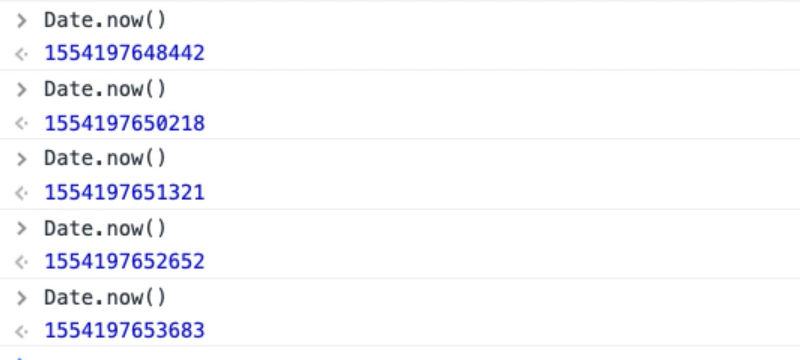Remember this is in milliseconds, so if I actually create a todo within the same millisecond we'll have possibly the same problem as we did before, but my bot isn't going to run on a millisecond basis.

It's going to run every 500 milliseconds, 100 milliseconds...

So, I want to log this. Now, I might want to log the actual values, in which case I would put this in the string first, and then print out, but this will do fine for the moment.

Whilst I'm here let's create the amend.

In fact, I only want to do that if I have found a random item because I need to amend it. So, it says find a random item, if we've actually found one then log that we are going to amend it.

``````this.amendRandomTodo = function(){
x = getRandomItemIndex();
if(x>0){
console.log("amend todo" + x);
autoTodo.amendTodo(x, "amended random todo " +  Date.now());
}
}
``````

If I run this now, I have the ability to `rando.createRandomTodo`.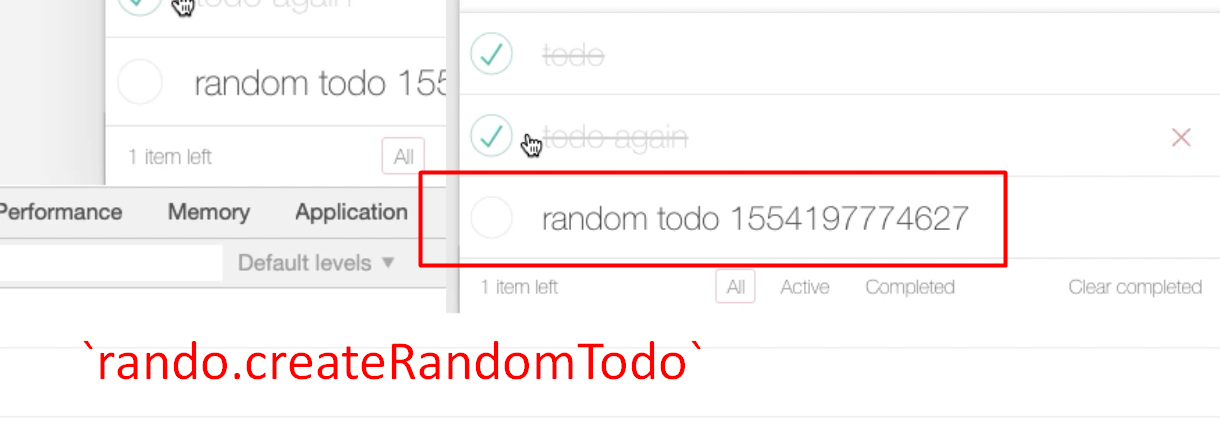Then `rando.amendRandomTodo`.

There we go. See how it's starting to amend them?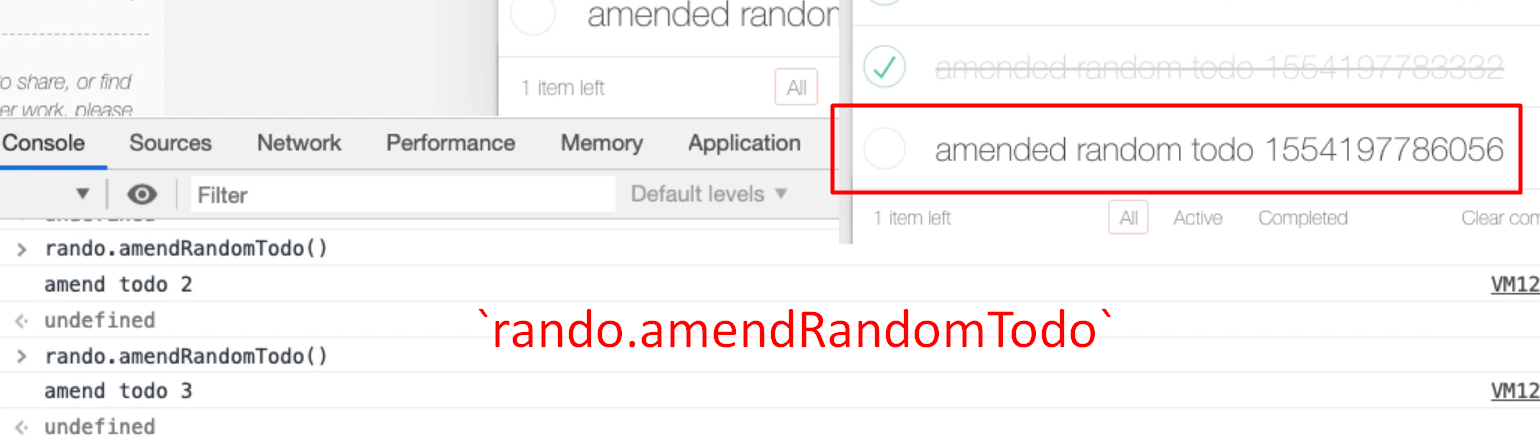You can see the timestamp on the end changing as it does so.

Now if I had a bot that had the ability to randomly loop around this, we'd have something that would interact with the application.

I'm just going to create a new snippet for that. Rename this to “random usage todo bot”.

We already essentially wrote the code that we need for this, so let me just find that in the console.

There we go. But this time rather than looping around `autoTodo` we're going to loop around `rando`.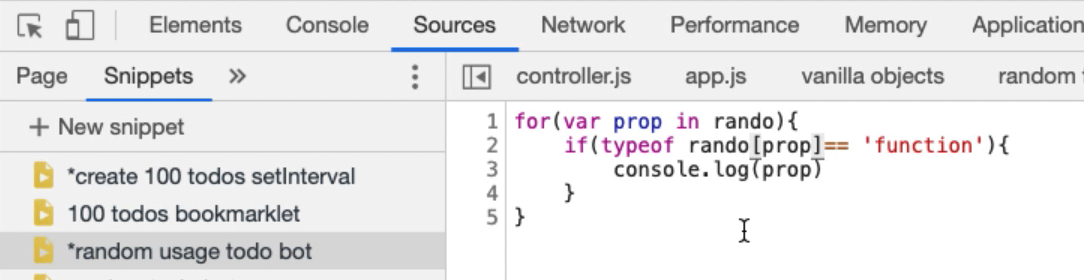If I run that in the Console you see the `toggleAll`, `selectRandomItem`, `createRandomTodo` and `amendRandomTodo`.

What I need to do then is instead of “console dot logging” this, put it in an array.

So, I say `theFunctions` is going to be an empty array to start with. But as I got through this array, I'll say — `theFunctions.push` — the actual value.

``````var theFunctions = [];

for(var prop in rando){
if(typeof rando[prop]== 'function'){
theFunctions.push(prop)
}
}
``````

Now I'm going to choose a random item in this array.

I have to times this by an upper limit, and the upper limit is `theFunctions.length`

``````var randomFunctionIndex = Math.floor(Math.random()*theFunctions.length);
``````

Now if I say — `theFunctions[randomFunctionIndex`]— it will find the function that we've chosen.

That on its own isn't good enough, I need to say `rando`, that particular function, and then I want to execute it.

``````var theFunctions = [];

for(var prop in rando){
if(typeof rando[prop]== 'function'){
theFunctions.push(prop)
}
}

var randomFunctionIndex = Math.floor(Math.random()*theFunctions.length);

rando[theFunctions[randomFunctionIndex]]();
``````

This should create an empty array, populate it with all the functions on the `rando` object, chose a random value, then call that random value to do something.

Let me just set a breakpoint on this so we can see how it works.

If I run this now you should see some random actions happening on the screen there.

We've created a little bot that will do the work.

Let me just see if I can get this in break point mode. So, if I put a break point here, and run this we should see it in action.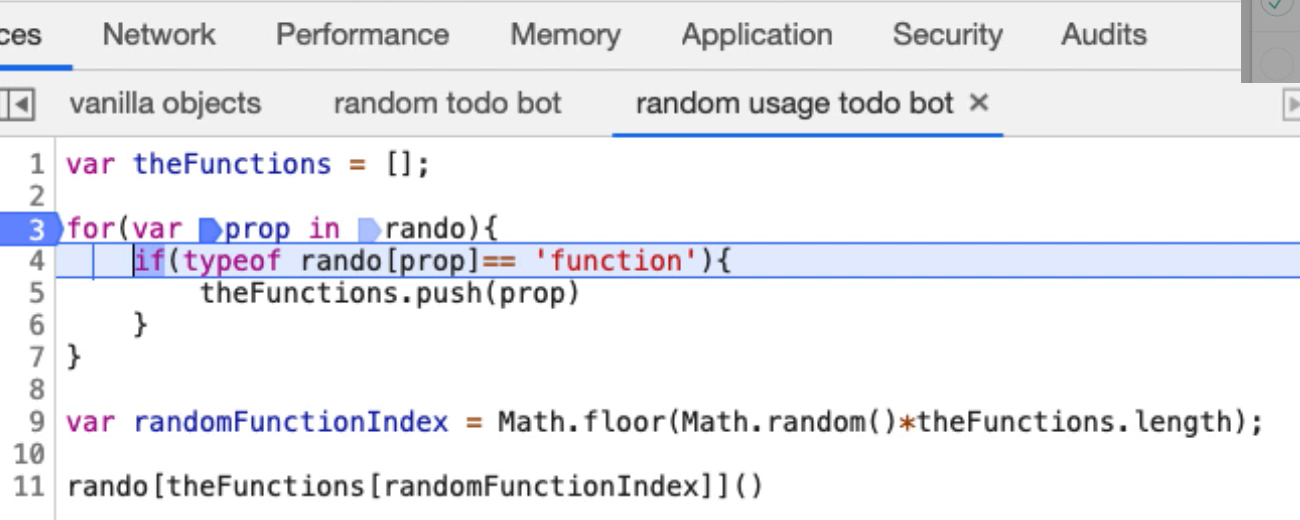So, what's happened is we've got `theFunctions`, and `theFunctions` is empty.

`rando` is an object with all these different properties, so we are going to loop around those.

If it has found one that is a function, and that's a function, it's going to add it into `theFunctions` array. So, `theFunctions` array now has `toggleAll`. These are just Strings in there. That's just the name.

So, now we've called the random code, which basically says chose a random value, times it by the length of `theFunctions` array which has got 4 things in it. Then `floor` it so it's an integer.

It’s given us the value 0, which is the first item.

So, then this is saying from `theFunctions` array chose the zero-indexed point, which is `theFunctions` - “toggleAll”.

Then this is basically saying call `rando` with `toggleAll` and execute that function.

So, if I Step Over this line now it should do a “toggle all”, which it does.

If I put this in a `setInterval` we'll have a bot that will randomly do things on the application.

Let me write the code for that.

The `setInterval` is going to be a `function`. The function contains this code and `setInterval` will happen every, let's say one second.

To stop this bot, I would have to write — `clearInterval(randoBot)` — so let me just add that as a comment to there remind me.

### # RandoBot - Random Usage Todo Bot Using SetInterval

``````var randoBot = setInterval(function(){

var theFunctions = [];

for(var prop in rando){
if(typeof rando[prop]== 'function'){
theFunctions.push(prop)
}
}

var randomFunctionIndex = Math.floor(Math.random()*theFunctions.length);

rando[theFunctions[randomFunctionIndex]]();

},1000);

//clearInterval(randoBot)
``````

Now if I run this, we're seeing a bunch of stuff happen. The log is coming through here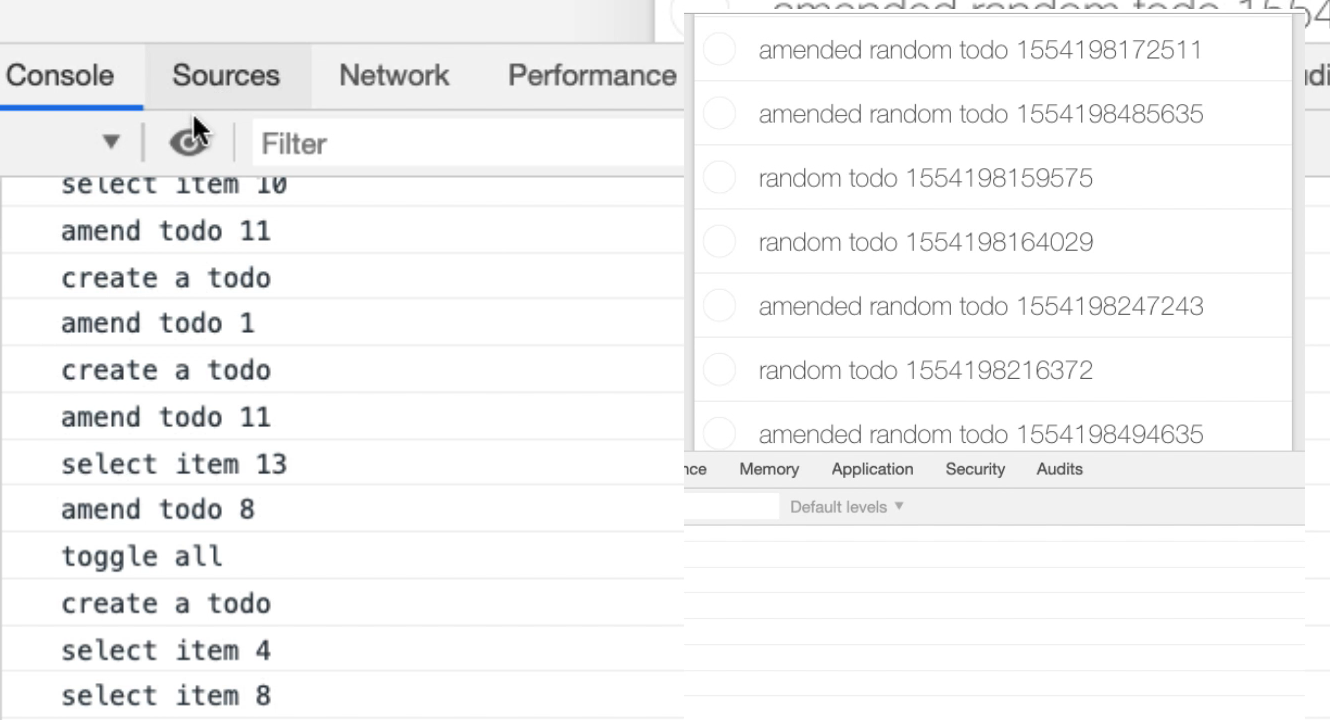Now I have something randomly interacting with the application. And I can stop that bot — `clearInterval(randoBot)` — there we go.

So, now I've written a little bot that will work.

The next thing I really have to do is just convert all the code in the `autoTodo` and create wrapper around for the `randoBot`.

I'm just going to go and do that, and when we come back, I'll show you what I've done.

Rando - Random Todo Bot

``````var rando = new function(){

function getRandomInt(x){
return Math.floor(Math.random() * x);
}

function getRandomItemIndex(){
max = document.querySelectorAll("ul.todo-list li").length;
if(max===0){
console.log("no items to choose from");
return 0;
}
x = getRandomInt(max)+1;
return x;
}

this.toggleAll = function(){
console.log("toggle all");
autoTodo.toggleAll();
}

this.selectRandomItem = function () {
x = getRandomItemIndex();
if(x>0){
console.log("select item " + x);
autoTodo.selectItemX(x);
}
}

this.deleteRandomItem = function () {
x = getRandomItemIndex();
if(x>0){
console.log("delete item " + x);
autoTodo.deleteItemX(x);
}
}

this.clearCompleted = function () {
console.log("clear completed");
autoTodo.clearCompleted();
}

this.filterAll = function () {
console.log("filter all");
autoTodo.filterAll();
}

this.filterActive = function () {
console.log("filter active");
autoTodo.filterActive();
}

this.filterCompleted = function () {
console.log("filter completed");
autoTodo.filterCompleted();
}

this.createRandomTodo = function(){
console.log("create todo");
autoTodo.createTodo("random todo " +  Date.now());
}

this.amendRandomTodo = function(){
x = getRandomItemIndex();
if(x>0){
console.log("amend todo" + x);
autoTodo.amendTodo(x, "amended random todo " +  Date.now());
}
}
}
``````

Okay. So, because you've seen all the patterns that were there before it should be fairly quick to run through this.

We have a `rando` object which has some private functions for controlling the random data generation that we've got in here.

All the other functions are essentially just calling the `autoTodo`.

• `toggleAll` is just a direct create a log, call the `toggleAll`.

• Select random item says chose a random item from the list then use `autoTodo` to select that item.

• `deleteRandomItem` is the same as `selectRandomItem`. It says chose an item from the list, if there is something in the list then use `autoTodo` to delete that item from the list.

• `clearCompleted` just is a wrapper around `autoTodo`.

• `filterCompleted` is a wrapper around `autoTodo`.

• `filterAll` is a wrapper around `autoTodo`.

• filterActive`is a wrapper around`autoTodo`.

• `createRandomTodo`, you saw me create that. It basically just says create a random name using the current timestamp, and call `autoTodo` to create a to do.

• `amendRandomTodo` is chose an item from the list then generate a random name for it.

And that's it.

Now because of the way that I wrote the “random usage todo bot” [`randoBot`] I don't have to change this because I can add more functions in the `rando` object, and this will just pick them up.

That was one of the benefits of doing it this way.

So, if I run the `randoBot` now it should be able to use most of the functionality in this application.

Eventually we'll see it clear it everything down, and let's get the console log up so we can see everything that it's doing.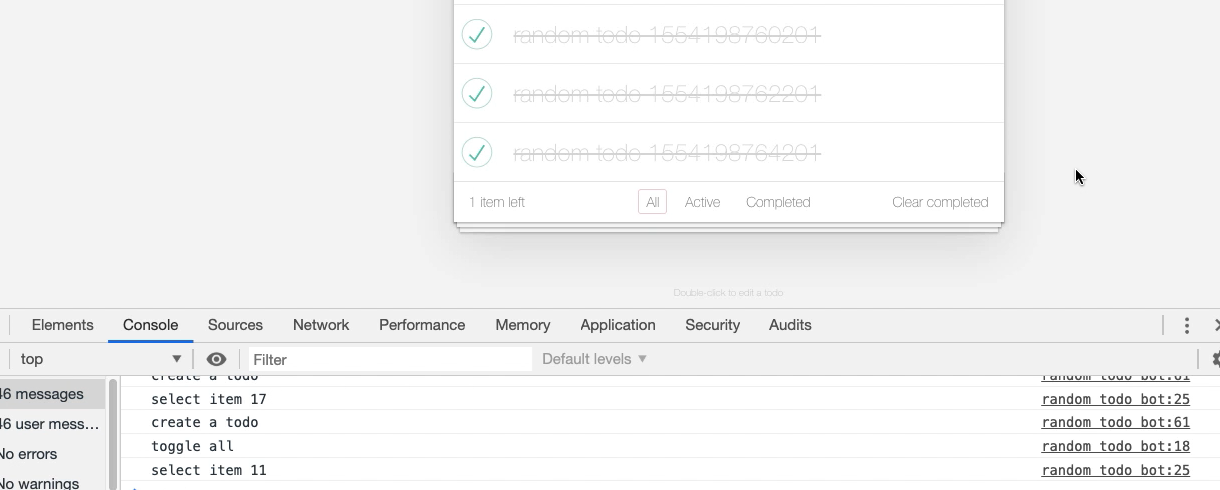So, it's selecting items, it's amending them. Selecting them, it's creating a to do. Creating another to do, amended it. Created a to do. So, it's now iterating through. We've got an automated execution.

This is actually model based testing.

What we've done is we've created a model of the application, which is this `randoTodoBot`.

All the functions that we can do in the application have been modeled in this `rando` object. Then we have something that will traverse that model. A coverage strategy, an execution strategy for that model.

In this case it's just randomly going to pick one of the functions, and choose it, and now we've got it running.

Let me close this bot down for a second — `clearInterval(randoBot)` — one of the reasons we add the `Interval` variable is that we can close the bot.

I'm going to just make this run a little bit faster so we can see it [changing time to 500 milliseconds].

Now if we were going to get any memory leaks, this might be a useful of testing that because we can leave this running for a while. Eventually the browser might start to explode.

If we really wanted to push it, we could get rid of any of the functions that actually clear it down and delete items.

Now one of the things I'm finding interesting here is I'm not seeing a lot of deletion. I'm not seeing a lot of clearing completed, so I'm not convinced it's actually working properly.

That's a hint.

Watching for Cues – Importance of Refresh

The hint there is I didn't refresh my bot when I hit play. It's very important that when we make changes to our code, we hit the refresh.

Now when I run this, we should see a much more. There we go.

So, now we have a bot that is going to clear things down.

Here we can see an issue with the model that we've created.

Because everything has the same probability, we may not get the same result out of our bot, right? It will keep deleting everything. It will keep clearing down everything that's deleted.

If we wanted to create a bot that pushed it from memory, we may not want to have it access the clear completed, or the delete functionality.

• I could make my execution strategy a bit more clever and only add in here functionality that I want.

• Or I could set up the functions to be the functions I know I want to create.

• Or when I add them into the array, instead of adding them into the array, I can add an object that has a probability with them, so that it executes the probability.

At the moment I've created a very naive model execution strategy.

Again, this is what you do to experiment. Once you have the bot you can leave it running, see if you get the results that you want, and then just change the execution strategy.

The important thing is knowing the basic strategy.

The basic strategy is our bots are going to be running in a set interval. If we have complicated application functionality, we create objects that will help us. Very often I'll put these in the same snippet, so I run them all at the same time.

You have seen the basics of all the techniques that I use for automating applications within the browser. I've used this to automate applications, to create helper tools for creating data, for creating executions so it can in parallel as I'm doing other testing, for creating completely autonomous game play bots that will run against an application.

This just is a massively useful extension of the console, and not many people do this. So, this is a good thing to experiment with.